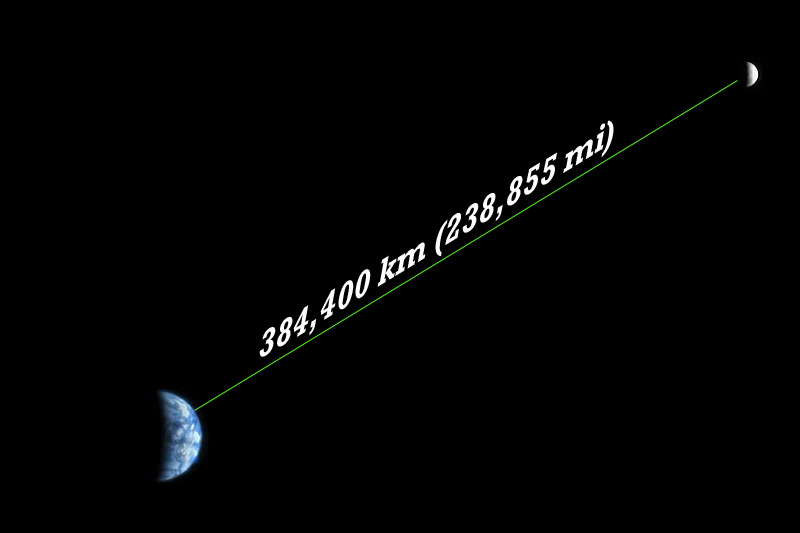In astronomy, the distance of the Moon from Earth is known as the lunar distance. The average distance of the Moon from the center of the Earth is calculated to be 384,400 km (238,855 mi). This is the average of the distance at the apogee and perigee of the Moon from Earth.

Methods for Calculation

The first person to scientifically calculate the distance of the Moon from Earth is Hipparchus in 2nd century BC. He used the methods of trigonometry to calculate the distance. His measurement was about 26,000 km (16,156 mi) off the right distance. The error he made was about 6.8%. There were other mathematical calculations which were more accurate. These mathematical calculations were made with the help of reference points. This was done by choosing the fixed stars as reference points.

More accurate measurements were made by high precision instruments which can calculate the time taken by light to travel from Earth to the Moon. A laser beam is sent from the LIDAR (Light Detection and Ranging) station on Earth. This light beam is reflected back to Earth by retroreflectors placed on the surface of the Moon. With this setup it is able to calculate the distance of the Moon from Earth with a precision of millimeters.

Actual Distance of Moon from Earth

The Moon is not always at a constant distance from the Earth. At times the Moon comes closer to Earth and at times it is further away. The closest position of the Moon is called lunar perigee and the distance at that time is calculated to be 356,700 km (221,643 mi). When the Moon is away from Earth, it is called apogee. At apogee, the distance of the Moon from Earth is 406,300 km (252,463 mi). The average of these two distances gives the actual Lunar Distance, 384,400 km (238,855 mi). The time taken by light to travel this distance is 1.26 seconds. Lunar distance has some significance in astronomy. Near Earth Object Catalog of NASA gives the distance of comets and asteroids in Lunar Distances.